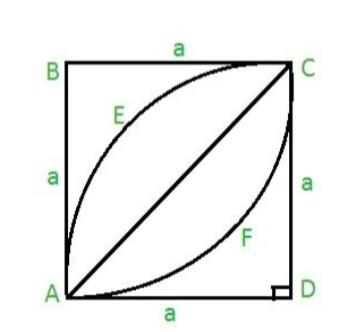# Area of a leaf inside a square in C Program?

To find the area of leaf inside a square, we need to split it into parts and find the area of parts and then add the areas to find the area of leaf.

To calculate the area we are splitting the leaf into two parts.

To find the area of 1st part AECA, we will find the area of quad circle AECDA and subtract the area of triangle ACDA from it.area of a Quadrant = 1⁄4 *(π*r2) where

π = 22/7 or 3.141.

area of a right angle triangle= 1⁄2*B*H = ½ a2

## Example

#include
#define PI 3.14159265
int main() {
float a = 12.3;
float area= a * a * (PI / 2 - 1);
printf("area = %f", area);
return 0;}

## Output

area = 86.355782